﻿ 航行体水下排气的热特征及影响因素分析
 舰船科学技术2020, Vol. 42Issue (1): 51-55PDF

Research on thermal characteristics and influencing factors of underwater exhaust of submersible
YUAN Jiang-tao, WANG Kun, SUN Bin, ZHANG Xin
Naval Submarine Academy, Qingdao 266199, China
Abstract: Aiming at the problem of underwater exhaust heat exposure of submersibles, the mathematical calculation model was established by Euler-Lagrange method. Based on the computational fluid mechanics and the computational heat transfer method, the changes of the exhaust gas temperature, the submersibles speed, exhaust gas flow rate and the temperature of the sea water on the thermal characteristic were analyzed. Results show that it is advantaged for the submersibles by reducing the exhaust gas flow rate, enhancing the submersibles speed, reducing the exhaust gas temperature. The thermal characteristic of underwater exhaust gas is not sensible to the changes of the sea water temperature.
Key words: multiphase flow     exhaust     thermal characteristics     influences
0 引　言

1 数学模型

 $\frac{{\partial \left( {\rho {u_i}\phi } \right)}}{{\partial {x_j}}} = \frac{\partial }{{\partial {x_j}}}\left( {{\varGamma _\phi }\frac{{\partial \phi }}{{\partial {x_j}}}} \right) + {S_\phi } + {S_{p\phi }}{\text{。}}$ (1)

 \left\{ \begin{aligned} & {N_k} = \mathop \smallint \nolimits {n_k}{v_{kn}}{\rm d}A = {\rm{const}}{\text{，}}\\ & \frac{{{\rm d}{v_{ki}}}}{{{\rm d}t}} = \frac{{\left( {{v_i} - {v_{ki}}} \right)}}{{{\tau _{rk}}}} + {g_i} + \left( {{v_i} - {v_{ki}}} \right)\frac{{{{\dot m}_k}}}{{{m_k}}}{\text{，}}\\ & \frac{{{\rm d}{T_k}}}{{{\rm d}t}} = \frac{{\left[ {{{\dot m}_k}\left( {{c_p}T - {c_k}{T_k}} \right) - {Q_k}} \right]}}{{\left( {{m_k}{c_k}} \right)}}{\text{。}} \end{aligned} \right. (2)

2 数值模拟 2.1 计算模型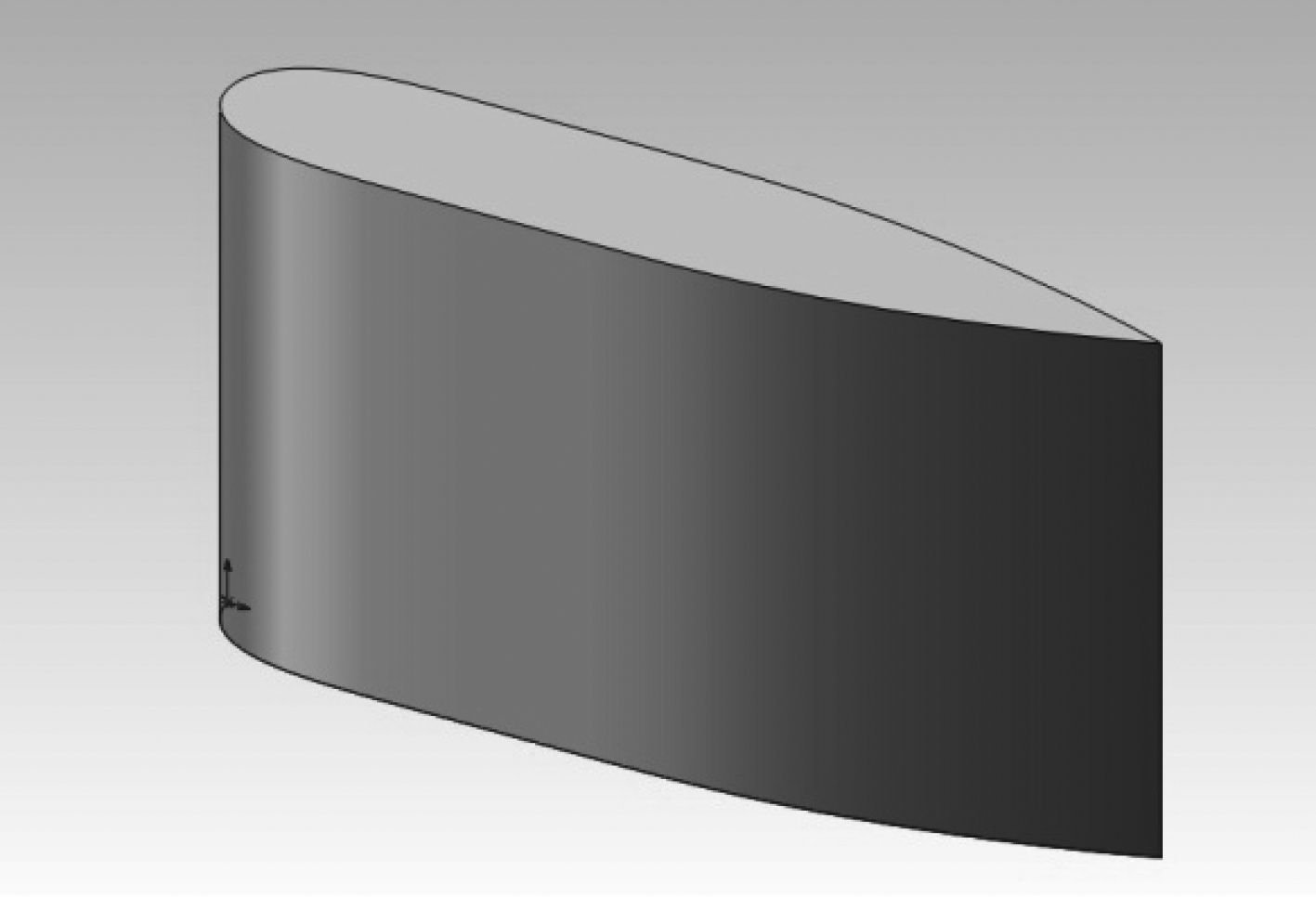图 1 航行体指挥台围壳三维模型 Fig. 1 3D model of superstructure hull
2.2 计算区域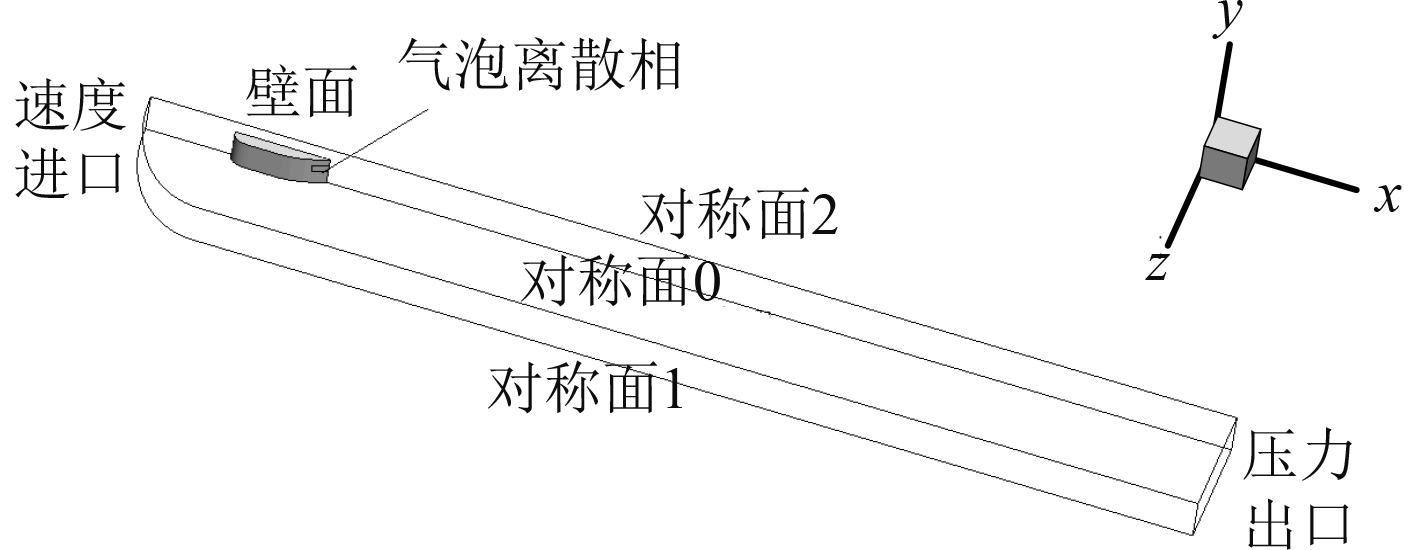图 2 计算区域 Fig. 2 Calculation region
2.3 辅助条件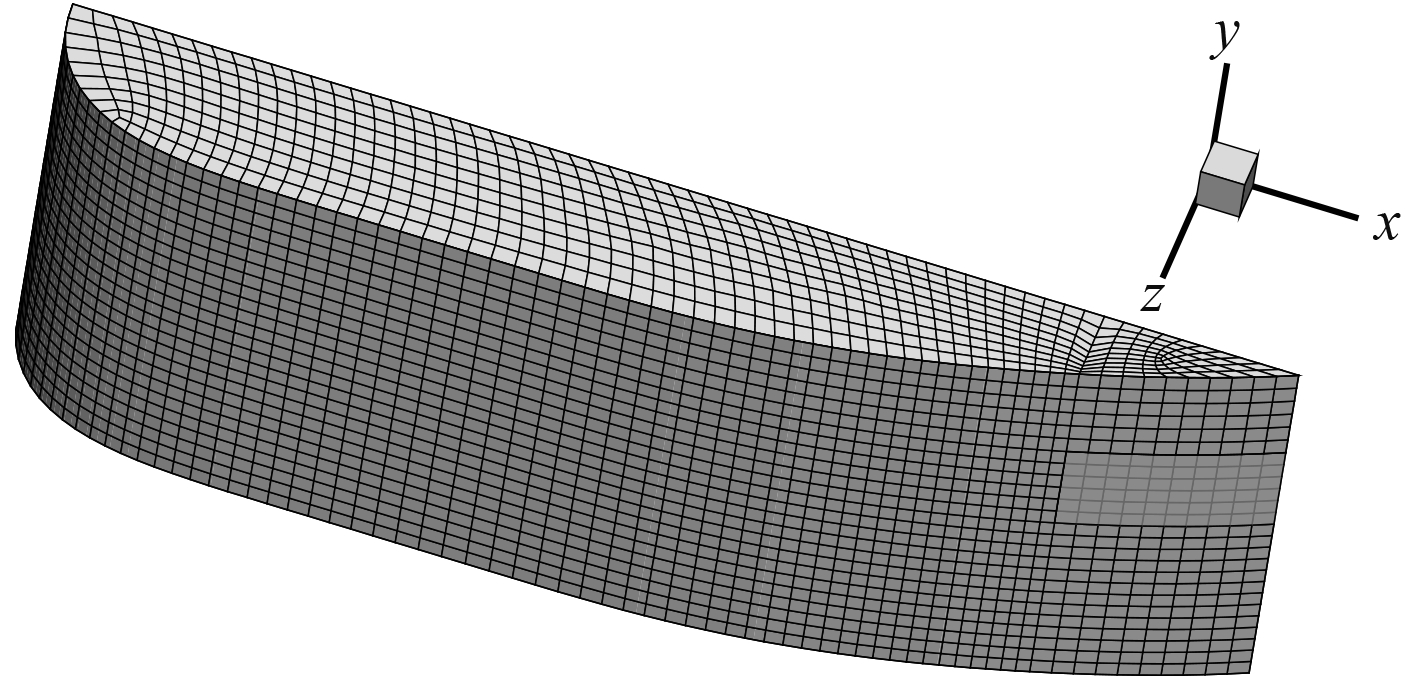图 3 围壳表面网格及离散相边界条件 Fig. 3 Shell surface mesh and boundary conditions
2.4 模型验证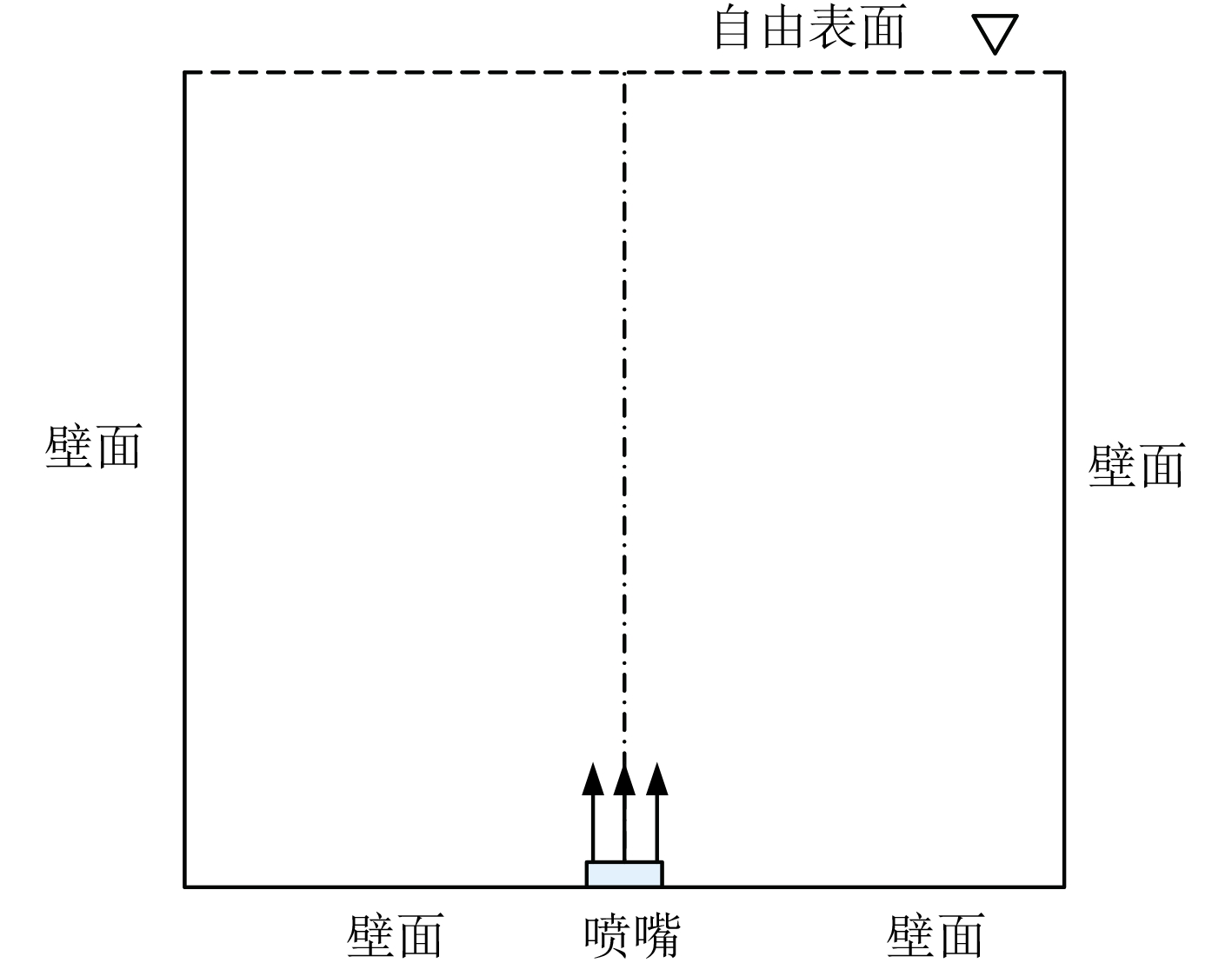图 4 计算区域及边界示意图 Fig. 4 Calculation region and boundaries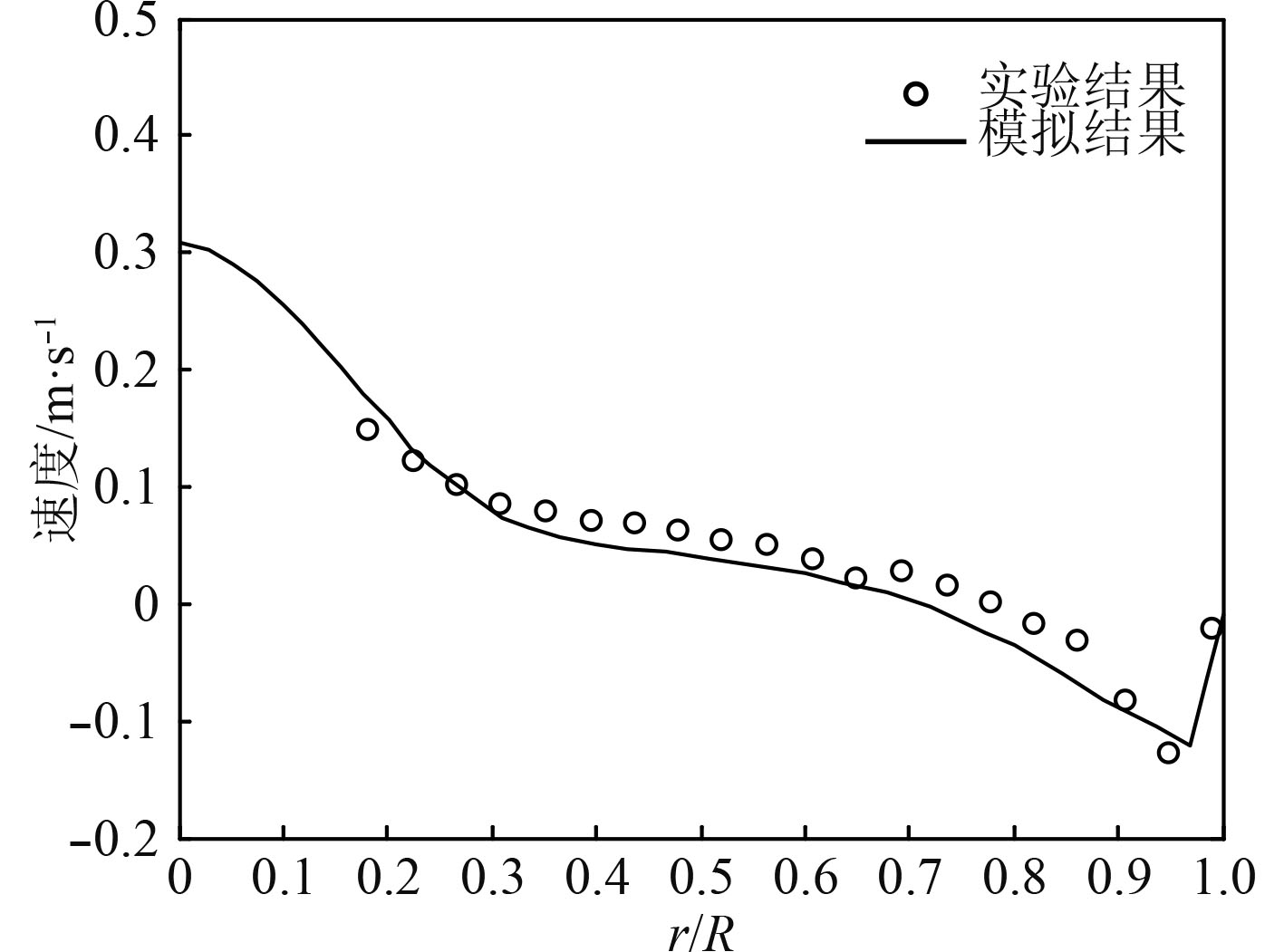图 5 液相速度模拟结果与实验结果的比较 Fig. 5 Simulated and experimental values of liquid phase velocity
3 结果分析

3.1 排气温度的影响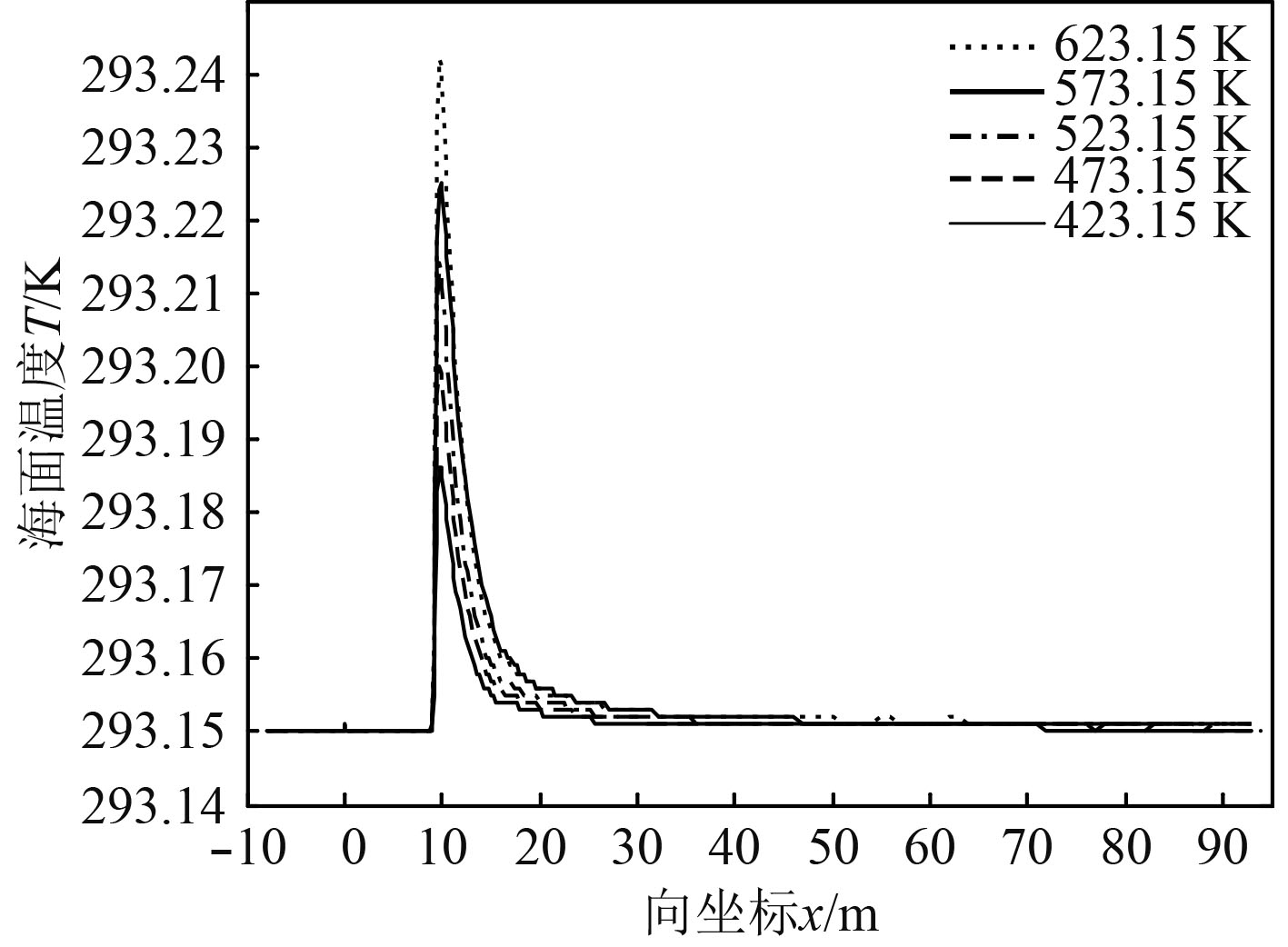图 6 不同排气温度时海面温度分布 Fig. 6 Effect of exhaust temperature on sea surface temperature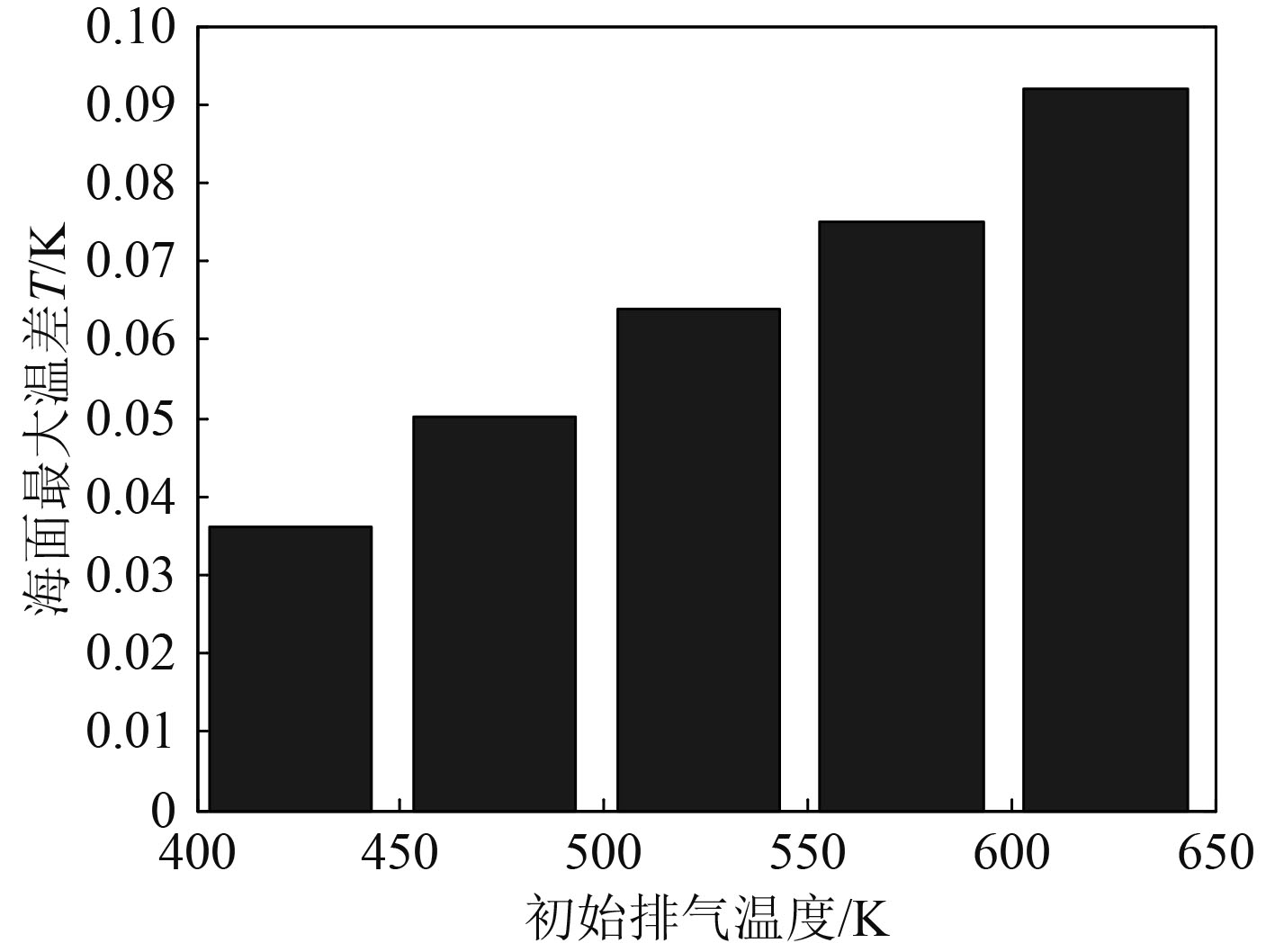图 7 不同排气温度下的海面最大温差 Fig. 7 Sea surface MAX temperature difference vs. exhaust temperature
3.2 排气流量的影响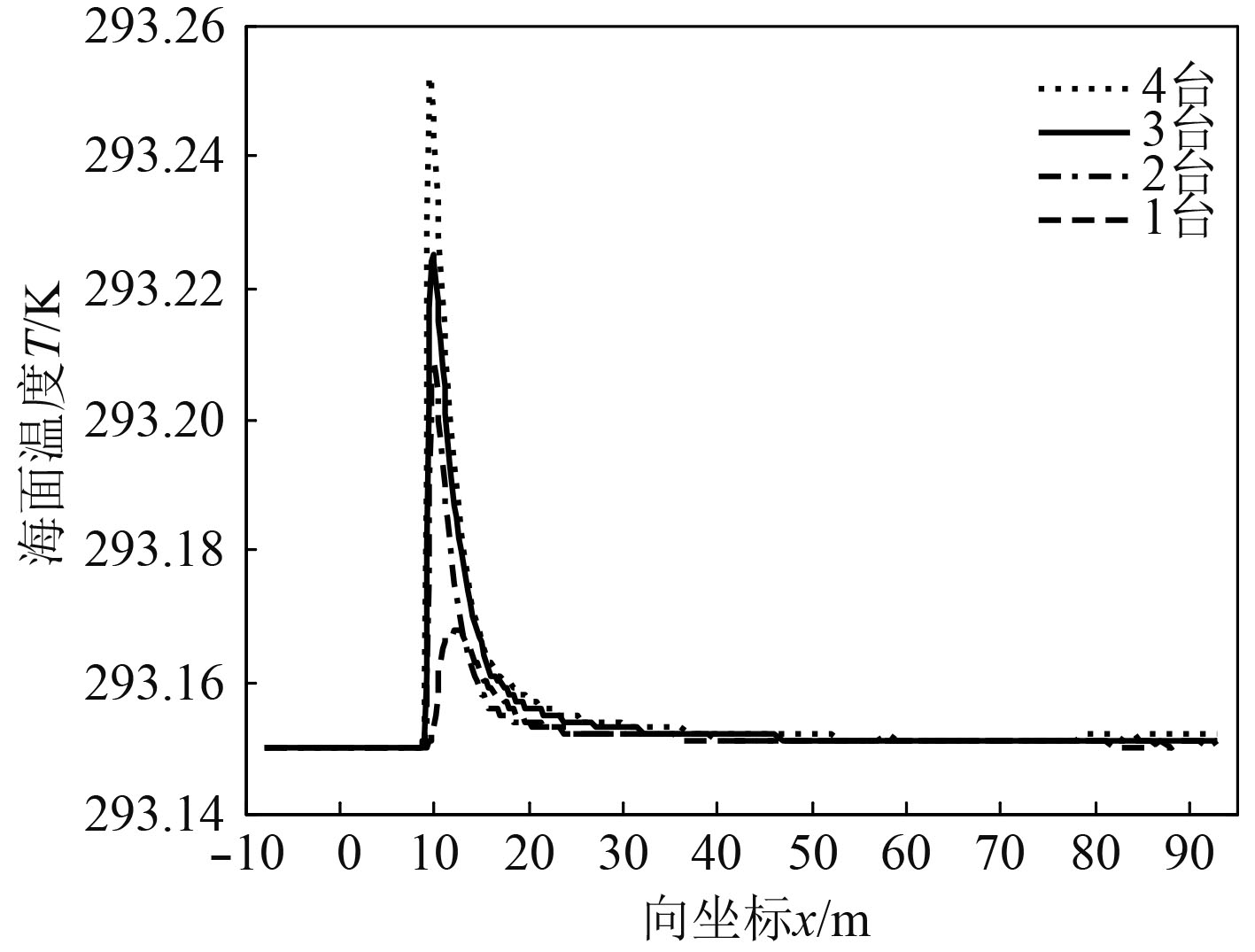图 8 不同排气量时海面温度分布 Fig. 8 Effect of exhaust displacement on sea surface temperature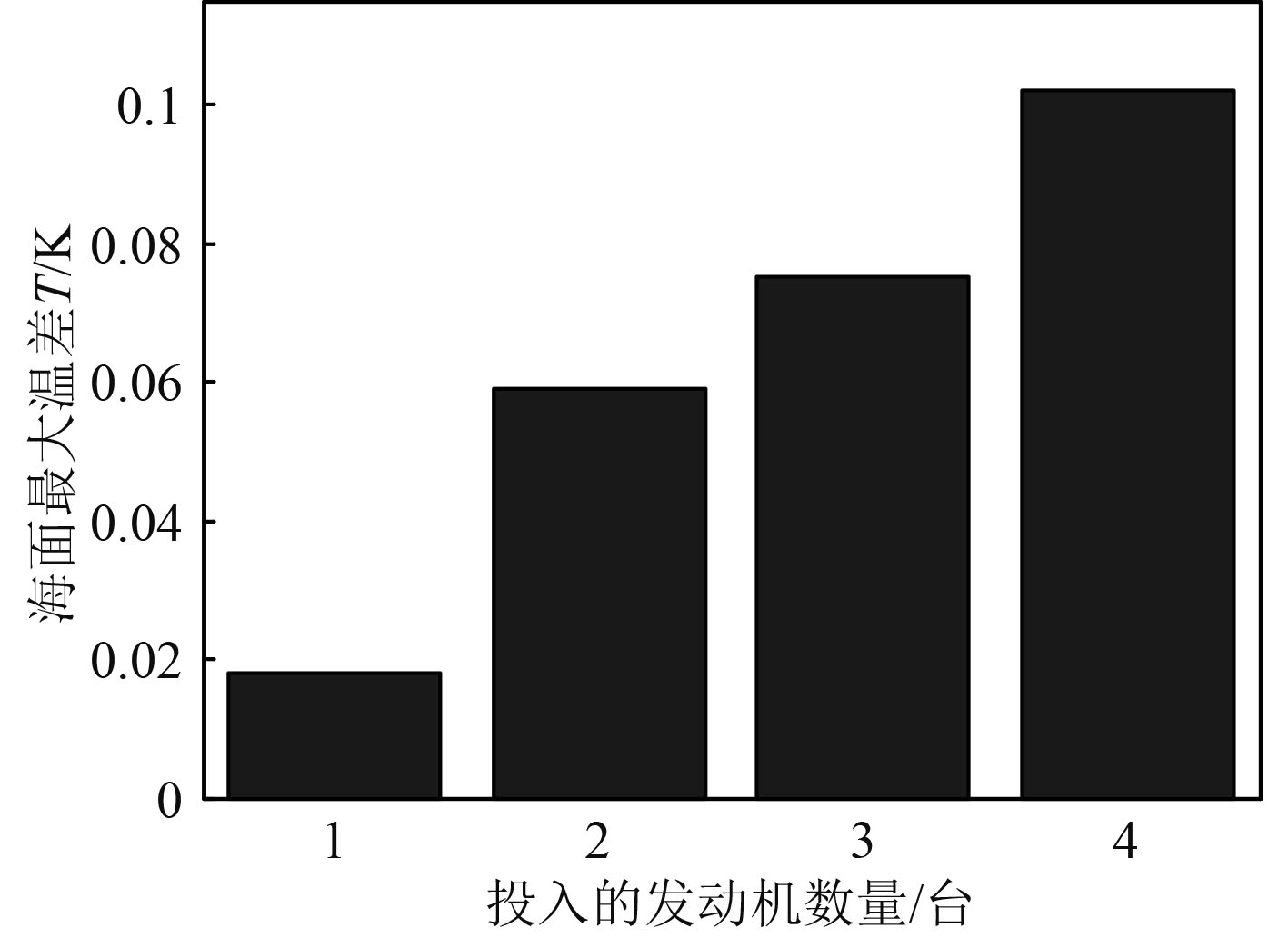图 9 不同排气量下的海面最大温差 Fig. 9 Sea surface MAX temperature difference vs. exhaust displacement
3.3 海水温度的影响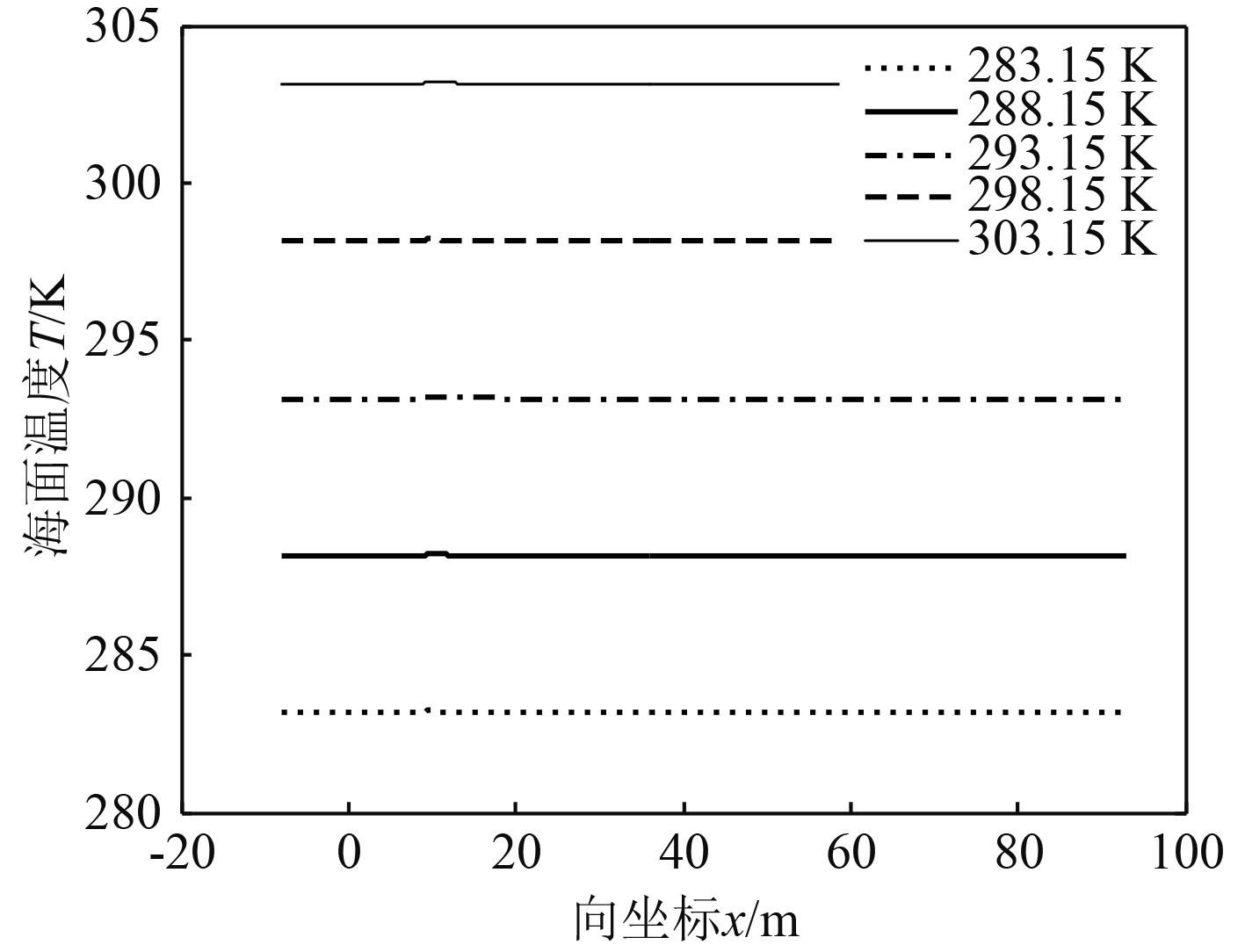图 10 不同海水温度下海面的温度分布 Fig. 10 Effect of sea temperature on sea surface temperature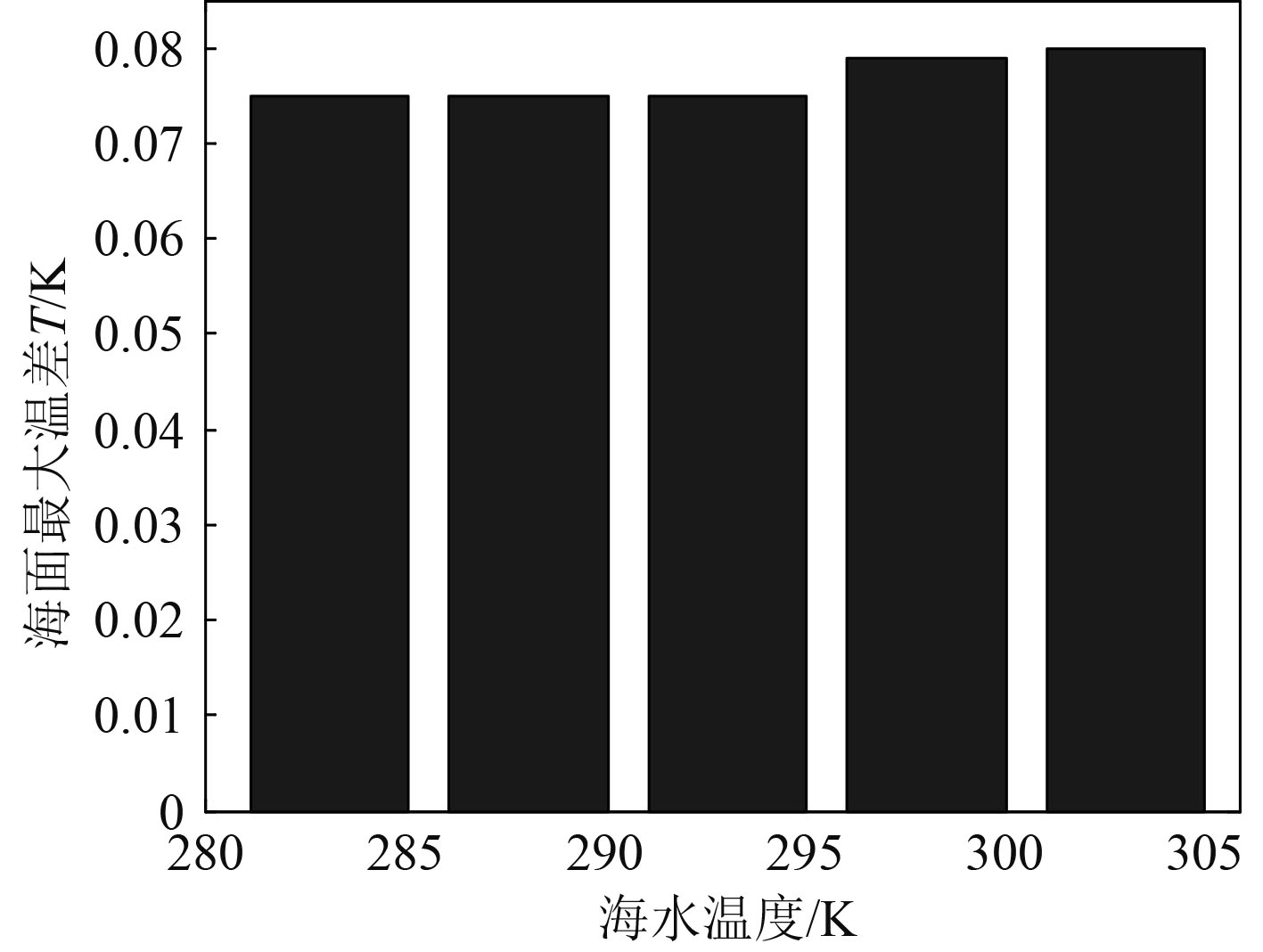图 11 不同海水温度下海面最大温差 Fig. 11 Sea surface MAX temperature difference vs. sea temperature
3.4 航行速度的影响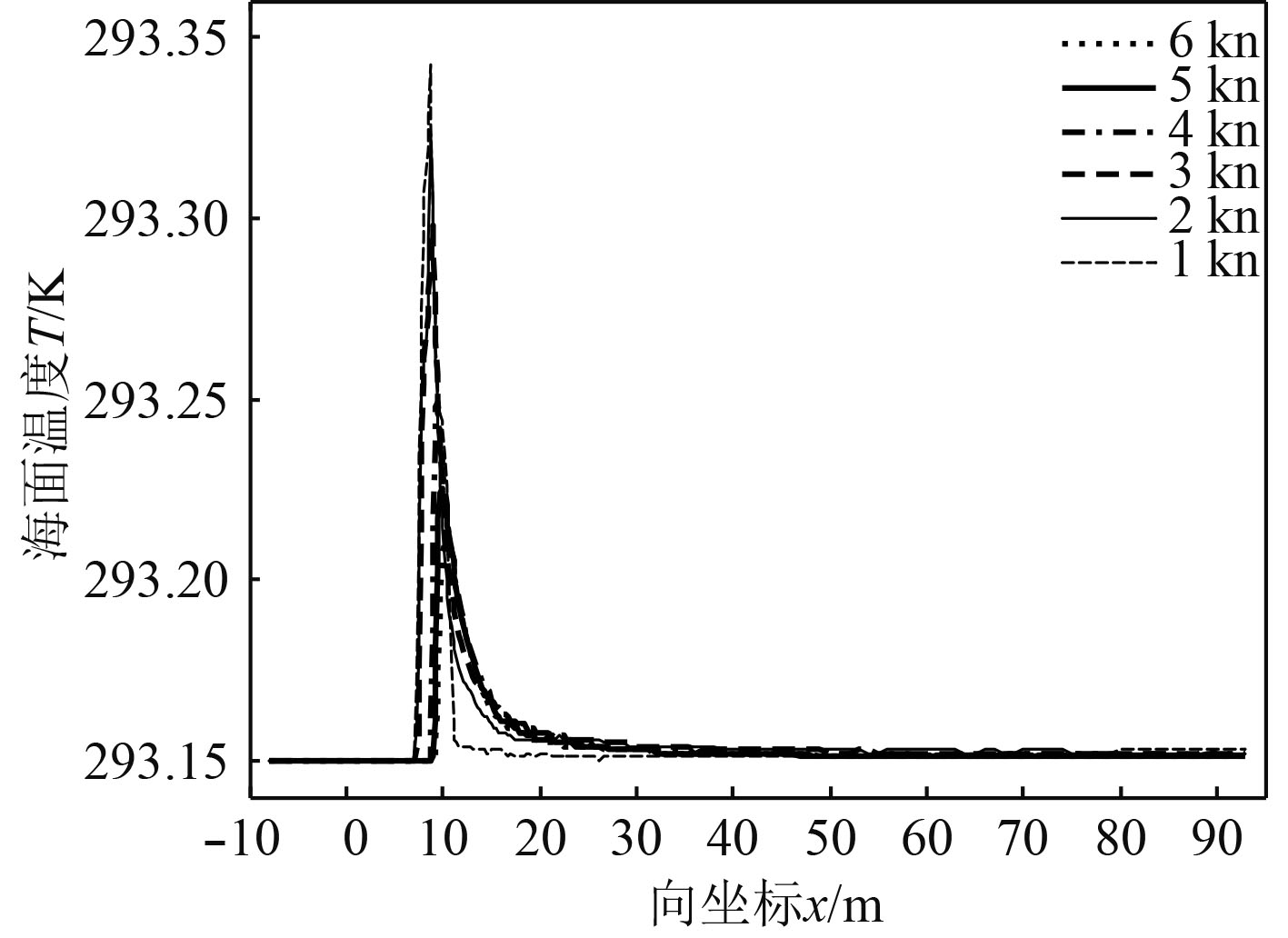图 12 不同航速下的温度分布 Fig. 12 Effect of submersible speed on sea surface temperature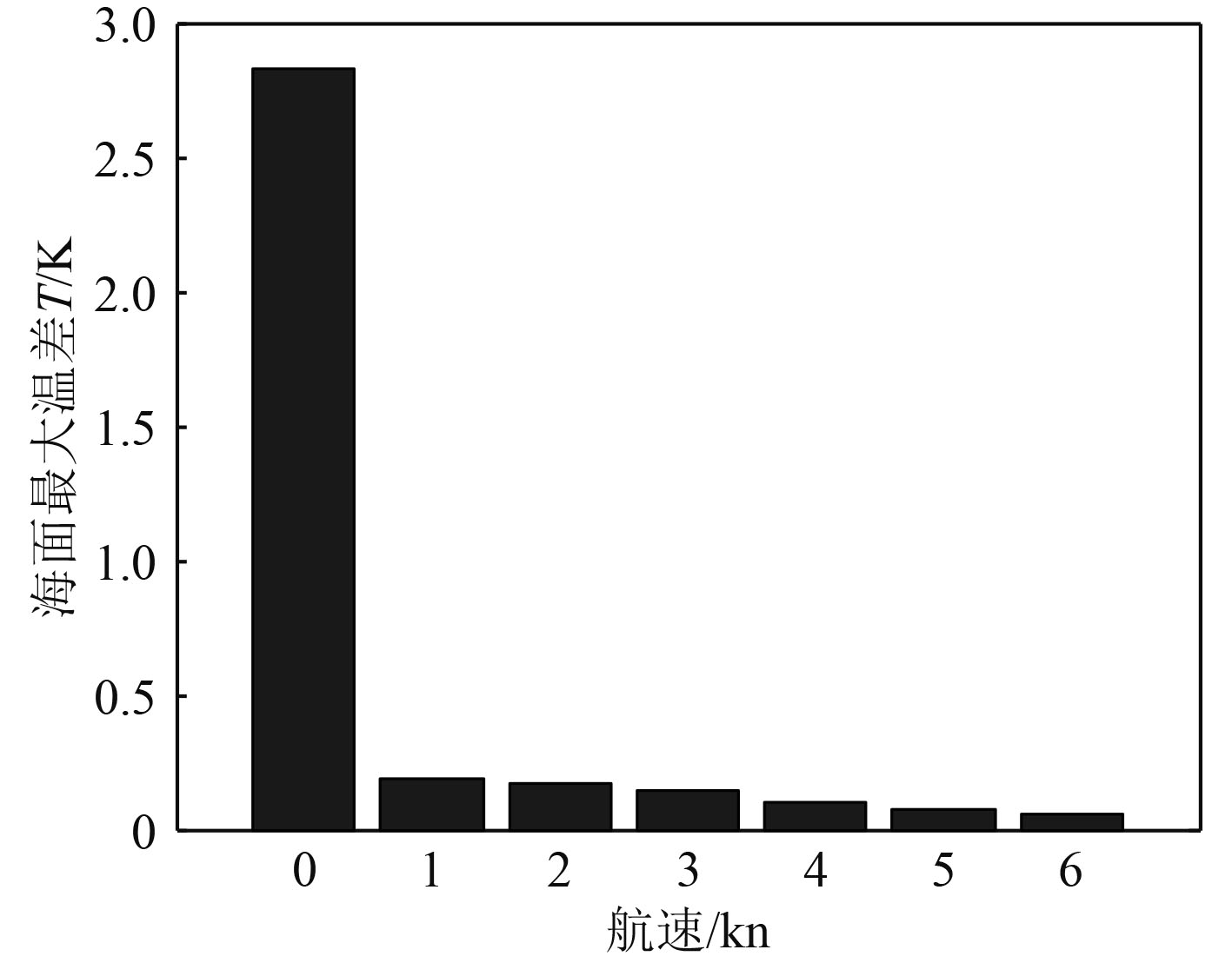图 13 不同航速下的海面最大温差 Fig. 13 Sea surface MAX temperature difference vs. submersible speed
4 结　语

  鲁新平, 沈振康. 红外热成像系统应用于反潜探测的分析[J]. 红外与激光工程, 2002, 31(3): 217-219, 236. DOI:10.3969/j.issn.1007-2276.2002.03.007  张建奇, 方小平. 红外物理 [M]. 西安: 西安电子科技大学出版社, 2004.  张建生, 孙传东, 卢迪. 水中气泡的特性研究[J]. 西安工业学院学报, 2000, 20(1): 1-8. DOI:10.3969/j.issn.1673-9965.2000.01.001  李帅, 孙龙泉, 张阿漫. 水中上浮气泡动态特性研究[J]. 物理学报, 2014, 63(18): 287-299.  吴凤林, G Tsang. 关于气泡羽流的研究[J]. 水动力学研究与进展, 1989, 4(1): 104-112.  马霞, 李国栋, 高扬, 等. 不同曝气量和纵横比下针孔喷射气泡羽流摆动特性的数值模拟研究[J]. 水动力学研究与进展A辑, 2016, 31(4): 433-440.  王治云, 李永胜, 杨茉. 水下排气两相流动及气泡粒径分布的数值模拟[J]. 能源工程, 2017(6): 49-52.  鲍文春, 权晓波, 魏海鹏. 航行体排气水下发射流体动力数值仿真研究[J]. 导弹与航天运载技术, 2014(5): 14-18.  周力行. 多相湍流反应流体力学 [M]. 北京: 国防工业出版社, 2002.  Fluent Inc. Fluent 6.3 user’s guide [Z]. Lebanon, USA: Fluent Inc, 2006.  GROVES N C, HUANG T T, CHANG M S. Geometric Characteristics of DARPA SUBOFF Models[R]. Bethesda, MD: David Taylor Research Center, 1989.  卢云涛. 全附体潜艇的流场和流噪声的数值研究 [D]. 上海: 上海交通大学, 2008. http://cdmd.cnki.com.cn/article/cdmd-10248-2008052695.htm  DOMGIN J F, GARDIN P. Experimental and numeriacl investigation of gas stirred ladles [C]//M Brunet. Second International Conference on CFD in the Minerals and Process Industries. Melbourne, Austrilia: CSIRO, 1999.